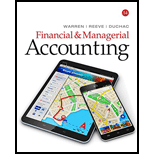# What are the principal objections to the use of the average rate of return method in evaluating capital investment proposals?### Financial & Managerial Accounting

14th Edition
Carl Warren + 2 others
Publisher: Cengage Learning
ISBN: 9781337119207### Financial & Managerial Accounting

14th Edition
Carl Warren + 2 others
Publisher: Cengage Learning
ISBN: 9781337119207

#### Solutions

Chapter
Section
Chapter 25, Problem 1DQ
Textbook Problem

## What are the principal objections to the use of the average rate of return method in evaluating capital investment proposals?

Expert Solution
To determine

Average Rate of Return:

Average rate of return is a method that measures the average earnings of a particular business, as a percentage of the average investment. It is also known as accounting rate of return.

Calculation of Average rate of return:

AverageRateofReturn}=(EstimatedAverageAnnualIncome)(AverageInvestment)×100

The principal objection to use the average rate of return method.

### Explanation of Solution

The major objection for using the average rate of return method is as follows:

1. Average rate of return method doesn’t consider the expected cash flows from the investment proposals.

2. It also doesn’t consider the period (timing) of the expected cash flows.

### Want to see more full solutions like this?

Subscribe now to access step-by-step solutions to millions of textbook problems written by subject matter experts!

Get Solutions

### Want to see more full solutions like this?

Subscribe now to access step-by-step solutions to millions of textbook problems written by subject matter experts!

Get Solutions